# Complete construction

Construct triangle ABC if hypotenuse c = 7 cm and angle ABC = 30 degrees. / Use Thales' theorem - circle /. Measure and write down the length of legs.

Result

a =  6.062 cm
b =  3.5 cm

#### Solution:Try calculation via our triangle calculator.Leave us a comment of example and its solution (i.e. if it is still somewhat unclear...):

Showing 0 comments:Be the first to comment!#### To solve this example are needed these knowledge from mathematics:

See also our right triangle calculator. See also our trigonometric triangle calculator.

## Next similar examples:

1. Triangle SSAConstruct a triangle ABC if |AB| = 5cm va = 3cm, CAB = 50 °. It is to create the analysis and construction steps.
2. Construct 1Construct a triangle ABC, a = 7 cm, b = 9 cm with right angle at C, construct the axis of all three sides. Measure the length of side c (and write).
3. Ruler and compassUse a ruler and compass to construct a triangle ABC with AB 5cm BAC 60° and ACB 45°.
4. Circle tangentIt is given to a circle with the center S and radius 3.5 cm. Distance from the center to line p is 6 cm. Construct a circle tangent n which is perpendicular to the line p.
5. Outer contact of circles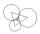Construct a circle k1 (S1; 1.5 cm), k2 (S2; 2 cm), and K3 (S3; 2.5 cm) so that they are always two outer contact. Calculate the perimeter of the triangle S1S2S3.
6. Right triangleDraw a right triangle ABC if |AB| = 5 cm |BC| = 3 cm, |AC| = 4 cm. Draw Thales circle above the hypotenuse of the triangle ABC.
7. Tangents constructCircle is given k (S; 2.5 cm) and an outer line p. Construct a tangent t of the circle that has with a line p angle 60°. How many solutions has the task?
8. Regular octagonDraw the regular octagon ABCDEFGH inscribed with the circle k (S; r = 2.5 cm). Select point S' so that |SS'| = 4.5 cm. Draw S (S '): ABCDEFGH - A'B'C'D'E'F'G'H'.
9. Semicircle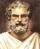In the semicircle with center S and the diameter AB is constructed equilateral triangle SBC. What is the magnitude of the angle ∠SAC?
10. OK circleCalculate the radius (circumradius) of the circle described right triangle with hypotenuse long 33 and one cathetus long 17.
11. The chordSide of the triangle inscribed in a circle is a chord passing through circle center. What size are the internal angles of a triangle, if one of them is 40°?
12. Inscribed triangle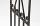To a circle is inscribed triangle so that the it's vertexes divide circle into 3 arcs. The length of the arcs are in the ratio 2:3:7. Determine the interior angles of a triangle.
13. The bridge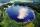Across the circle lakepasses through its center bridge over the lake. At three different locations on the lake shore are three fishermen A, B, C. Which of fishermen see the bridge under the largest angle?
14. Obtuse angle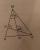The line OH is the height of the triangle DOM, line MN is the bisector of angle DMO. obtuse angle between the lines MN and OH is four times larger than the angle DMN. What size is the angle DMO? (see attached image)
15. RT - inscribed circleIn a rectangular triangle has sides lengths> a = 30cm, b = 12.5cm. The right angle is at the vertex C. Calculate the radius of the inscribed circle.
16. Chord MNChord MN of circle has distance from the center circle S 120 cm. Angle MSN is 64°. Determine the radius of the circle.
17. Center traverseIt is true that the middle traverse bisects the triangle?